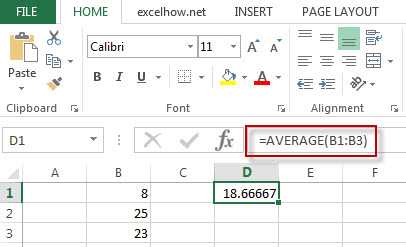# Excel Average Function

This post will guide you how to use Excel AVERAGE function with syntax and examples in Microsoft excel.

### Description

The Excel AVERAGE function returns the average of the numbers that you provided.

The AVERAGE function is a build-in function in Microsoft Excel and it is categorized as a Statistical Function.

The AVERAGE function is available in Excel 2016, Excel 2013, Excel 2010, Excel 2007, Excel 2003, Excel XP, Excel 2000, Excel 2011 for Mac.

### Syntax

The syntax of the AVERAGE function is as below:

`= AVERAGE (number1,[number2],…)`

Where the AVERAGE function arguments are:

• Number1 -This is a required argument.  the first numeric values
• Number2 – This is an optional argument.

### Example

The below examples will show you how to use Excel AVERAGE Function to return the average of the numbers provided.

#1 To get the average value of the numbers in cell range B1:B3 Cell, just using the following excel formula:

`=AVERAGE(B1:B3)`### Related Functions

• Excel MODE Function
The Excel MODE function returns the most frequently occurring number found in an array or range of numbers. If there are two or more frequently occurring numbers in a range of data, it will return the lowest of these numbers.The syntax of the MODE function is as below:= MODE(number1, [number2], …)…
• Excel MEDIAN Function
The Excel MEDIAN function returns the median of the given numbers. And the median is the number in the middle of a list of supplied numbers.  The syntax of the MEDIAN function is as below:= MEDIAN (number1, [number2], …)…